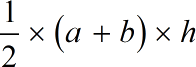Request a Tool

Online Trapezium Area Calculator

A quadrilateral with one pair of parallel sides is known as a trapezium. It is also defined in some circumstances as a flat figure having four sides, two of which are parallel.

Area
0

Formula• a = Parallel side 1 of the trapezium
• b = Parallel side 2 of the trapezium
• h = Distance between parallel sides

Defination / Uses

A rectangle with all sides equal, a rhombus with all angles equal, or a parallelogram with equal diagonals that bisect each other are all examples of trapeziums.

How to use trapeziums calcultor?

• Just add the values in their corresponding fields.